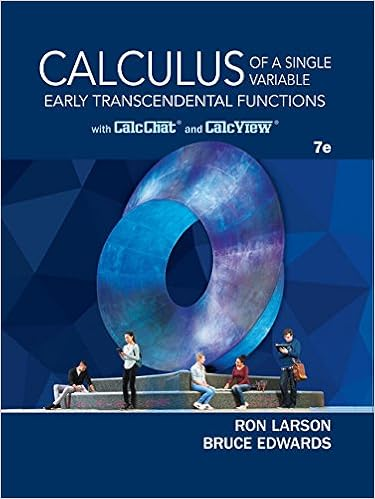2B-MultipleChoiceS17-posted_0.pdf - M ATH 2B MULTIPLE...

• 5

This preview shows page 1 - 3 out of 5 pages.

We have textbook solutions for you!
The document you are viewing contains questions related to this textbook.The document you are viewing contains questions related to this textbook.
Chapter 8 / Exercise 61
Calculus of a Single Variable: Early Transcendental Functions
Edwards/LarsonExpert Verified
M ATH 2B MULTIPLE CHOICE SAMPLE QUESTIONS , S PRING 2017 1. (Section 4.9) The function F ( x ) satisfies F 0 ( x ) = 3 x ( x - 2) and F (0) = 1 . What is F (1) ? a. -3 b. -3/2 c. -1 d. 0 e. 3/2
F IGURE 1. This shows the graph of a function f ( x ) referred to in Questions 2 and 3. 2. (Section 4.9) Let F ( x ) denote an antiderivative of f ( x ) , where y = f ( x ) is shown in Figure 1. Which of the following can we deduce about F ( - 5) ?
3. (Section 5.2) Figure 1 shows the graph of a function y = f ( x ) . Imagine we estimate both of the integrals R - 4 R 4 2 f ( x ) dx using Riemann sums with 20 rectangles and left endpoints. Which of the following is true?
We have textbook solutions for you!
The document you are viewing contains questions related to this textbook.The document you are viewing contains questions related to this textbook.
Chapter 8 / Exercise 61
Calculus of a Single Variable: Early Transcendental Functions
Edwards/LarsonExpert Verified
b. The estimate of R - 4 - 6 f ( x ) dx is an over-estimate and the estimate of R 4 2 f ( x ) dx is an under- estimate. c. The estimates of R - 4 - 6 f ( x ) dx and R 4 2 f ( x ) dx are both over-estimates. d. The estimates of R - 4 - 6 f ( x ) dx and R 4 2 f ( x ) dx are both under-estimates.
•••